This function calculates the break down algorithm for B random orderings. Then it calculates the distribution of attributions for these different orderings. Note that the shap() function is just a simplified interface to the break_down_uncertainty() function with a default value set to B=25.

break_down_uncertainty(x, ..., keep_distributions = TRUE, B = 10)

# S3 method for explainer
break_down_uncertainty(
x,
new_observation,
...,
keep_distributions = TRUE,
B = 10
)

# S3 method for default
break_down_uncertainty(
x,
data,
predict_function = predict,
new_observation,
label = class(x),
...,
path = NULL,
keep_distributions = TRUE,
B = 10
)

shap(x, ..., B = 25)

## Arguments

x

an explainer created with function explain or a model.

...

other parameters.

keep_distributions

if TRUE then we will keep distribution for predicted values. It's needed by the describe function.

B

number of random paths

new_observation

a new observation with columns that correspond to variables used in the model.

data

validation dataset, will be extracted from x if it is an explainer.

predict_function

predict function, will be extracted from x if it is an explainer.

label

name of the model. By default it's extracted from the 'class' attribute of the model.

path

if specified, then this path will be highlighed on the plot. Use average in order to show an average effect

## Value

an object of the break_down_uncertainty class.

break_down, local_attributions

## Examples

library("DALEX")
library("iBreakDown")
set.seed(1313)
model_titanic_glm <- glm(survived ~ gender + age + fare,
data = titanic_imputed, family = "binomial")
explain_titanic_glm <- explain(model_titanic_glm,
data = titanic_imputed,
y = titanic_imputed$survived, label = "glm") #> Preparation of a new explainer is initiated #> -> model label : glm #> -> data : 2207 rows 8 cols #> -> target variable : 2207 values #> -> predict function : yhat.glm will be used ( default ) #> -> predicted values : No value for predict function target column. ( default ) #> -> model_info : package stats , ver. 4.1.2 , task classification ( default ) #> -> predicted values : numerical, min = 0.1490412 , mean = 0.3221568 , max = 0.9878987 #> -> residual function : difference between y and yhat ( default ) #> -> residuals : numerical, min = -0.8898433 , mean = 4.198546e-13 , max = 0.8448637 #> A new explainer has been created! # there is no explanation level uncertanity linked with additive models bd_glm <- break_down_uncertainty(explain_titanic_glm, titanic_imputed[1, ]) bd_glm #> min q1 median mean #> glm: age = 42 -0.01492541 -0.01492541 -0.01434645 -0.01446344 #> glm: class = 3rd 0.00000000 0.00000000 0.00000000 0.00000000 #> glm: embarked = Southampton 0.00000000 0.00000000 0.00000000 0.00000000 #> glm: fare = 7.11 -0.01823177 -0.01784977 -0.01784977 -0.01773120 #> glm: gender = male -0.10843751 -0.10725651 -0.10725651 -0.10725810 #> glm: parch = 0 0.00000000 0.00000000 0.00000000 0.00000000 #> glm: sibsp = 0 0.00000000 0.00000000 0.00000000 0.00000000 #> glm: survived = 0 0.00000000 0.00000000 0.00000000 0.00000000 #> q3 max #> glm: age = 42 -0.01405996 -0.01396446 #> glm: class = 3rd 0.00000000 0.00000000 #> glm: embarked = Southampton 0.00000000 0.00000000 #> glm: fare = 7.11 -0.01741824 -0.01705077 #> glm: gender = male -0.10725293 -0.10667755 #> glm: parch = 0 0.00000000 0.00000000 #> glm: sibsp = 0 0.00000000 0.00000000 #> glm: survived = 0 0.00000000 0.00000000 plot(bd_glm)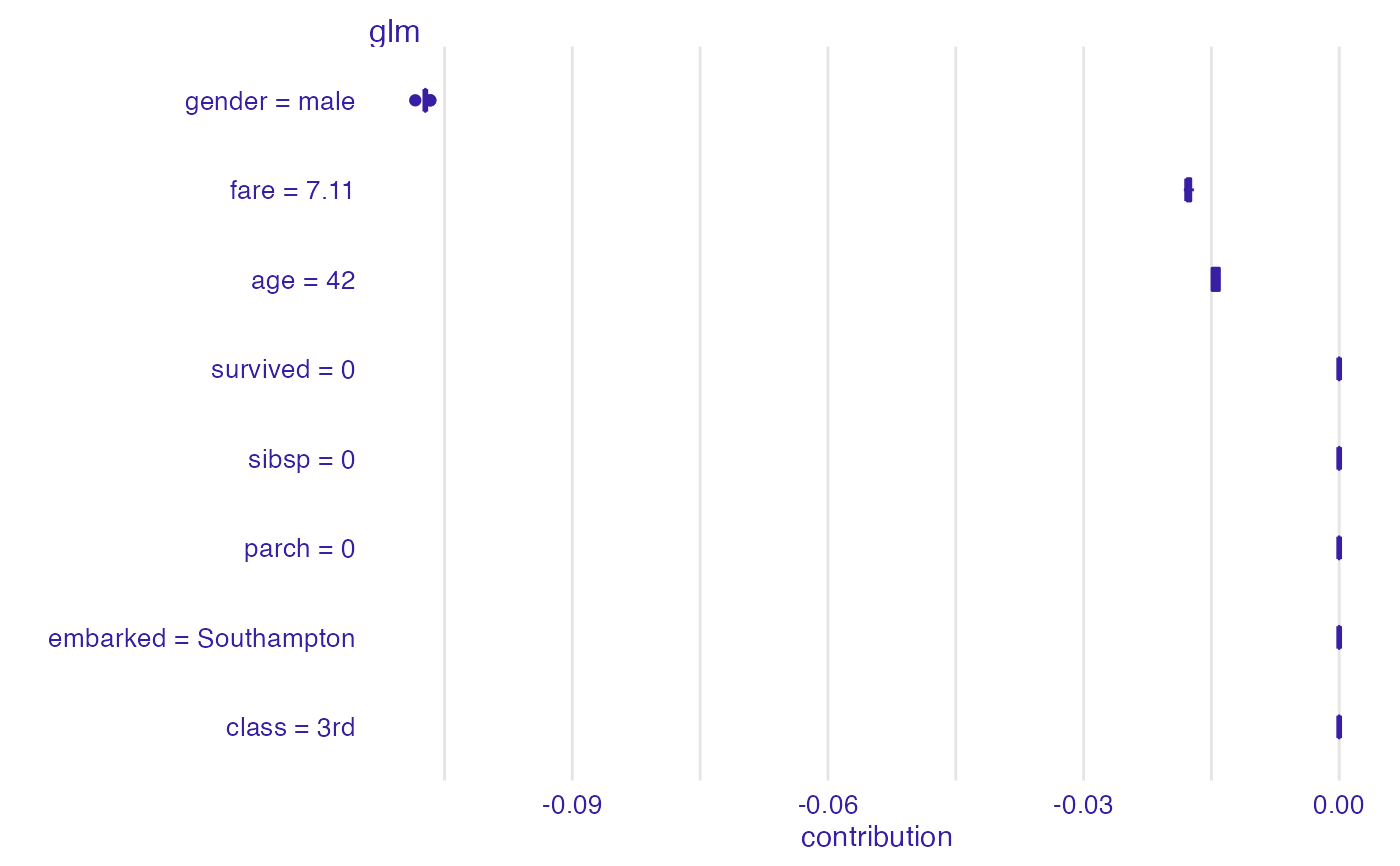# \dontrun{ ## Not run: library("randomForest") set.seed(1313) model <- randomForest(status ~ . , data = HR) new_observation <- HR_test[1,] explainer_rf <- explain(model, data = HR[1:1000, 1:5]) #> Preparation of a new explainer is initiated #> -> model label : randomForest ( default ) #> -> data : 1000 rows 5 cols #> -> target variable : not specified! ( WARNING ) #> -> predict function : yhat.randomForest will be used ( default ) #> -> predicted values : No value for predict function target column. ( default ) #> -> model_info : package randomForest , ver. 4.7.1 , task multiclass ( default ) #> -> model_info : Model info detected multiclass task but 'y' is a NULL . ( WARNING ) #> -> model_info : By deafult multiclass tasks supports only factor 'y' parameter. #> -> model_info : Consider changing to a factor vector with true class names. #> -> model_info : Otherwise I will not be able to calculate residuals or loss function. #> -> predicted values : predict function returns multiple columns: 3 ( default ) #> -> residual function : difference between 1 and probability of true class ( default ) #> A new explainer has been created! bd_rf <- break_down_uncertainty(explainer_rf, new_observation) bd_rf #> min q1 median mean #> randomForest.fired: age = 57.73 -0.021328 0.0247710 0.253395 0.1946016 #> randomForest.fired: evaluation = 2 -0.018856 0.0073270 0.032725 0.0216108 #> randomForest.fired: gender = male -0.009380 0.0054740 0.019250 0.0911182 #> randomForest.fired: hours = 42.32 0.167650 0.1953890 0.220689 0.2461712 #> randomForest.fired: salary = 2 -0.270298 -0.1751675 -0.160058 -0.1610878 #> randomForest.ok: age = 57.73 -0.346842 -0.3468420 -0.199269 -0.1834688 #> randomForest.ok: evaluation = 2 0.028666 0.1002960 0.125760 0.1215018 #> randomForest.ok: gender = male -0.282642 -0.1062540 -0.021756 -0.0845928 #> randomForest.ok: hours = 42.32 -0.106876 -0.0970580 -0.046824 -0.0447352 #> randomForest.ok: salary = 2 0.046824 0.1184785 0.118552 0.1311450 #> randomForest.promoted: age = 57.73 -0.126732 -0.0061320 -0.006132 -0.0111328 #> randomForest.promoted: evaluation = 2 -0.201822 -0.1749640 -0.166262 -0.1431126 #> randomForest.promoted: gender = male -0.045880 -0.0019940 -0.000019 -0.0065254 #> randomForest.promoted: hours = 42.32 -0.247972 -0.2398625 -0.189205 -0.2014360 #> randomForest.promoted: salary = 2 -0.003902 0.0069900 0.034329 0.0299428 #> q3 max #> randomForest.fired: age = 57.73 0.3529740 0.362800 #> randomForest.fired: evaluation = 2 0.0418870 0.045408 #> randomForest.fired: gender = male 0.1521340 0.280686 #> randomForest.fired: hours = 42.32 0.3072255 0.351330 #> randomForest.fired: salary = 2 -0.1390850 -0.070866 #> randomForest.ok: age = 57.73 -0.0178415 0.005860 #> randomForest.ok: evaluation = 2 0.1307830 0.196252 #> randomForest.ok: gender = male -0.0061560 -0.003480 #> randomForest.ok: hours = 42.32 0.0010015 0.030996 #> randomForest.ok: salary = 2 0.1542220 0.268992 #> randomForest.promoted: age = 57.73 0.0129425 0.015468 #> randomForest.promoted: evaluation = 2 -0.0957510 -0.058120 #> randomForest.promoted: gender = male 0.0023685 0.023564 #> randomForest.promoted: hours = 42.32 -0.1719955 -0.156930 #> randomForest.promoted: salary = 2 0.0415060 0.077562 plot(bd_rf)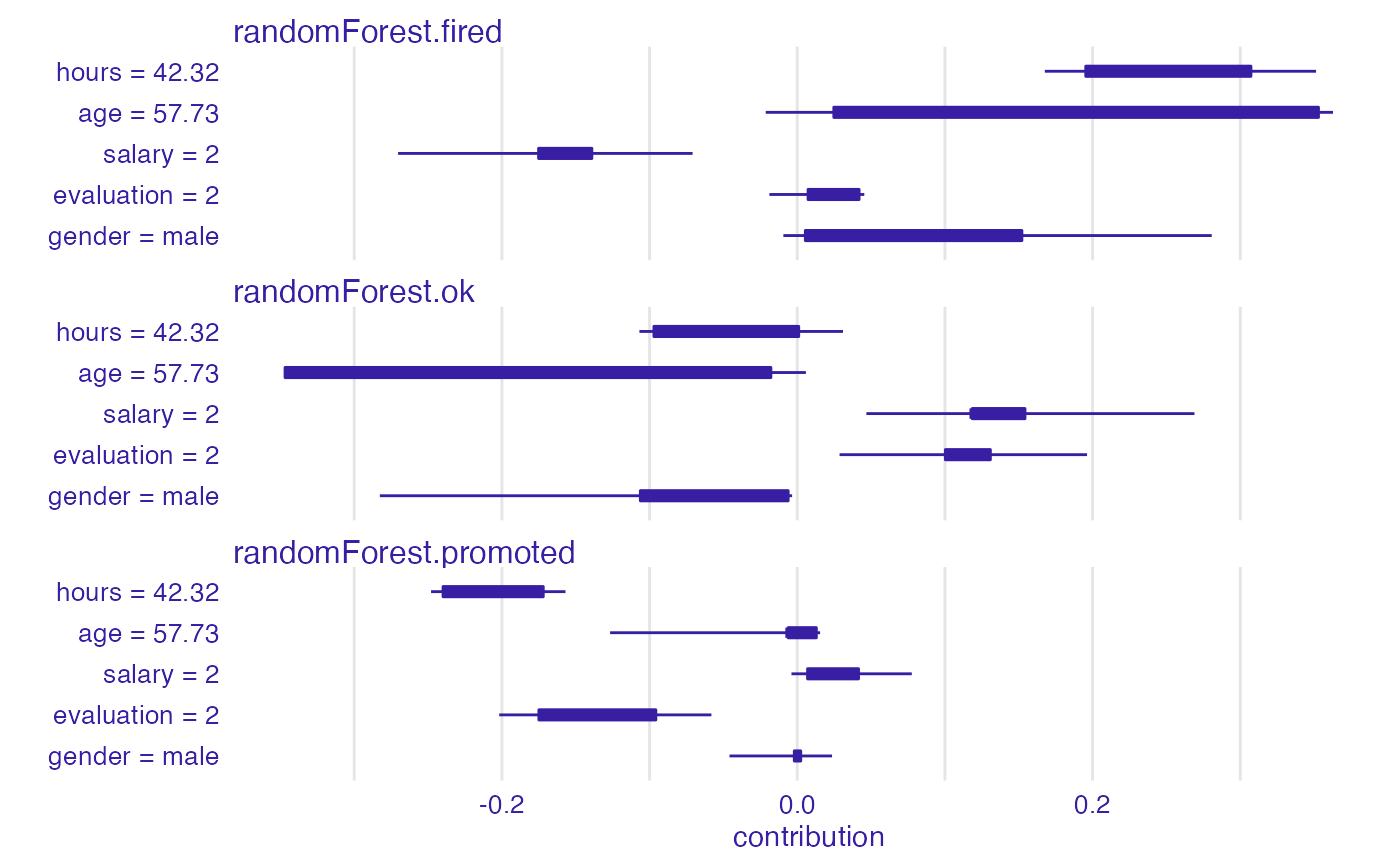# example for regression - apartment prices # here we do not have intreactions model <- randomForest(m2.price ~ . , data = apartments) explainer_rf <- explain(model, data = apartments_test[1:1000, 2:6], y = apartments_test$m2.price[1:1000])
#> Preparation of a new explainer is initiated
#>   -> model label       :  randomForest  (  default  )
#>   -> data              :  1000  rows  5  cols
#>   -> target variable   :  1000  values
#>   -> predict function  :  yhat.randomForest  will be used (  default  )
#>   -> predicted values  :  No value for predict function target column. (  default  )
#>   -> model_info        :  package randomForest , ver. 4.7.1 , task regression (  default  )
#>   -> predicted values  :  numerical, min =  2052.033 , mean =  3487.71 , max =  5776.623
#>   -> residual function :  difference between y and yhat (  default  )
#>   -> residuals         :  numerical, min =  -632.8469 , mean =  1.070017 , max =  1328.352
#>   A new explainer has been created!

bd_rf <- break_down_uncertainty(explainer_rf, apartments_test[1,])
bd_rf
#>                                              min        q1     median
#> randomForest: construction.year = 1976 -128.5908 -119.3910  -75.48837
#> randomForest: district = Srodmiescie    981.8193 1036.9753 1054.79081
#> randomForest: floor = 3                 178.8471  189.5230  194.12751
#> randomForest: no.rooms = 5             -229.8610 -225.7194 -212.31243
#> randomForest: surface = 131            -272.2211 -266.0785 -250.70512
#>                                              mean         q3        max
#> randomForest: construction.year = 1976  -82.87975  -50.06424  -47.64365
#> randomForest: district = Srodmiescie   1046.73182 1054.79081 1091.59037
#> randomForest: floor = 3                 197.65920  210.33113  215.52532
#> randomForest: no.rooms = 5             -200.17988 -203.34626 -130.21186
#> randomForest: surface = 131            -250.99715 -234.39585 -229.21426
plot(bd_rf)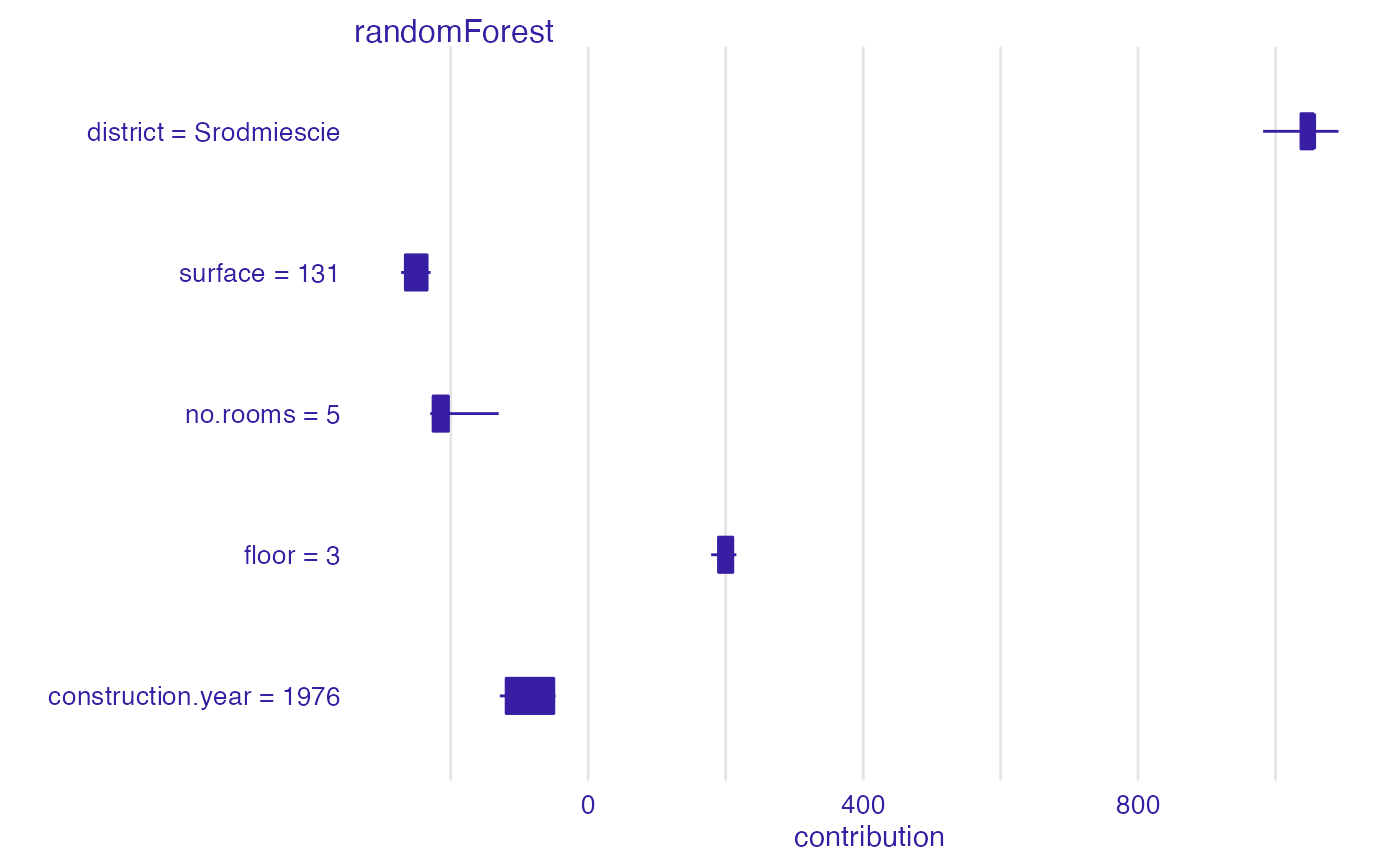bd_rf <- break_down_uncertainty(explainer_rf, apartments_test[1,], path = 1:5)
plot(bd_rf)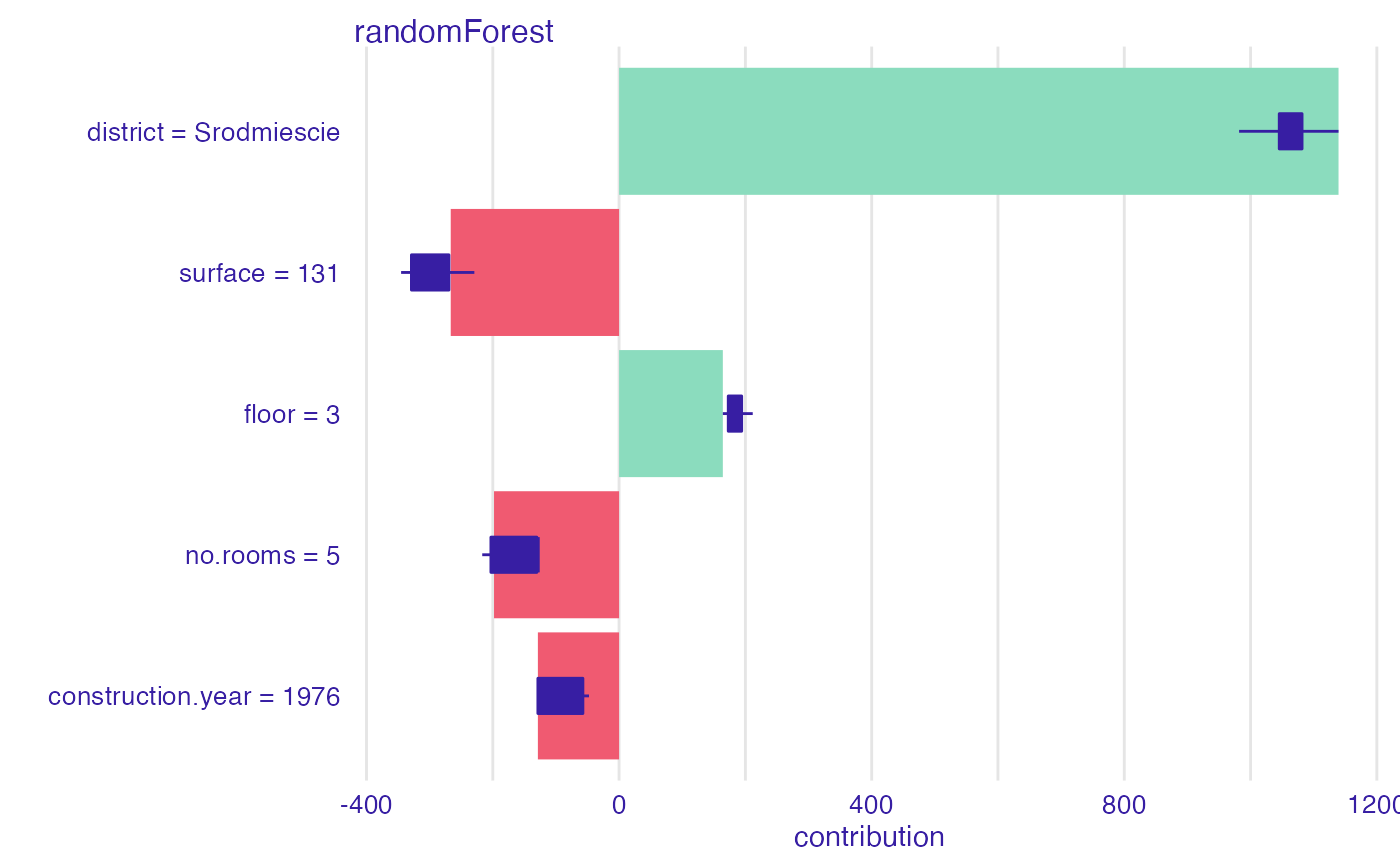bd_rf <- break_down_uncertainty(explainer_rf,
apartments_test[1,],
path = c("floor", "no.rooms", "district",
"construction.year", "surface"))
plot(bd_rf)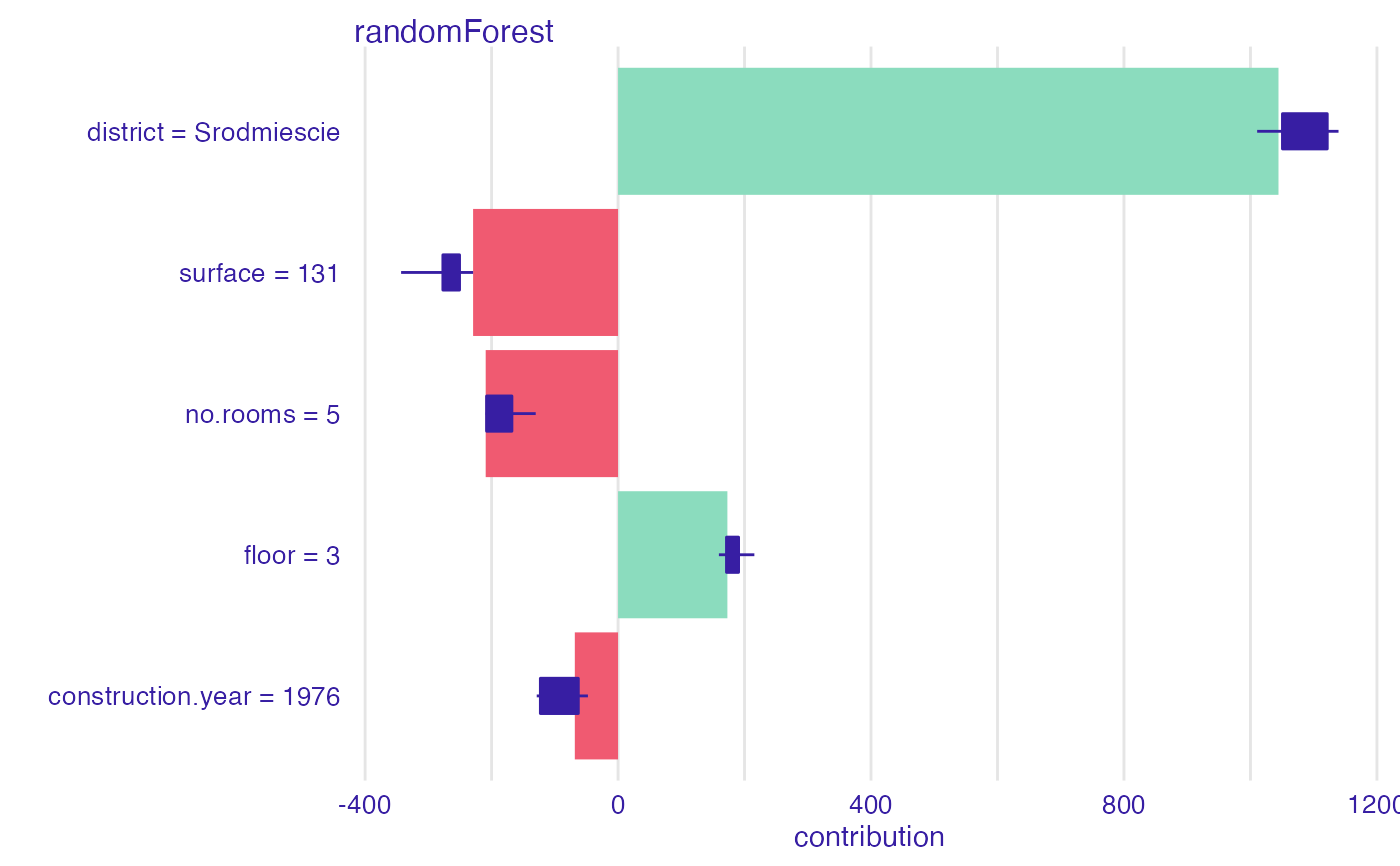bd <- break_down(explainer_rf,
apartments_test[1,])
plot(bd)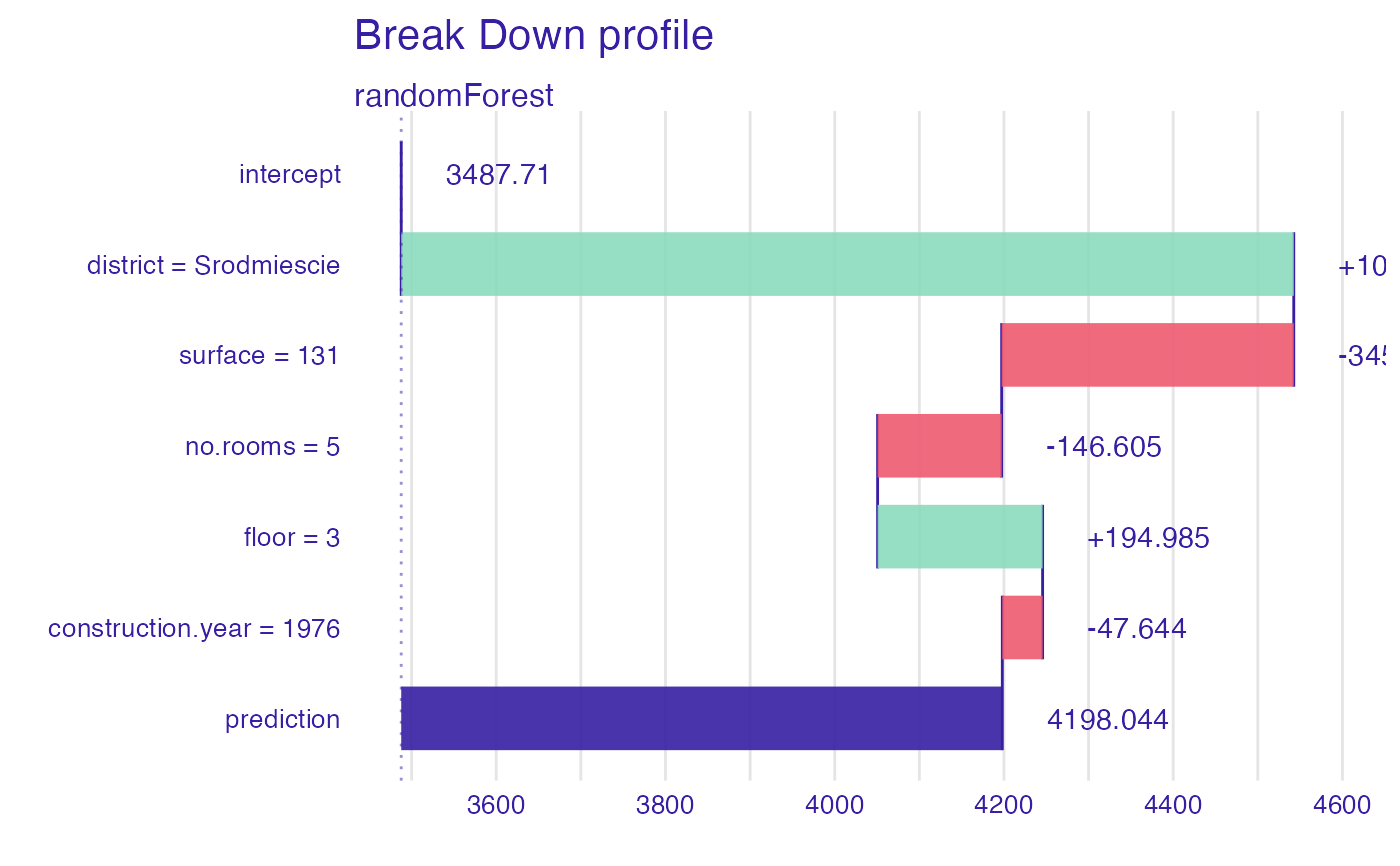s <- shap(explainer_rf,
apartments_test[1,])
plot(s)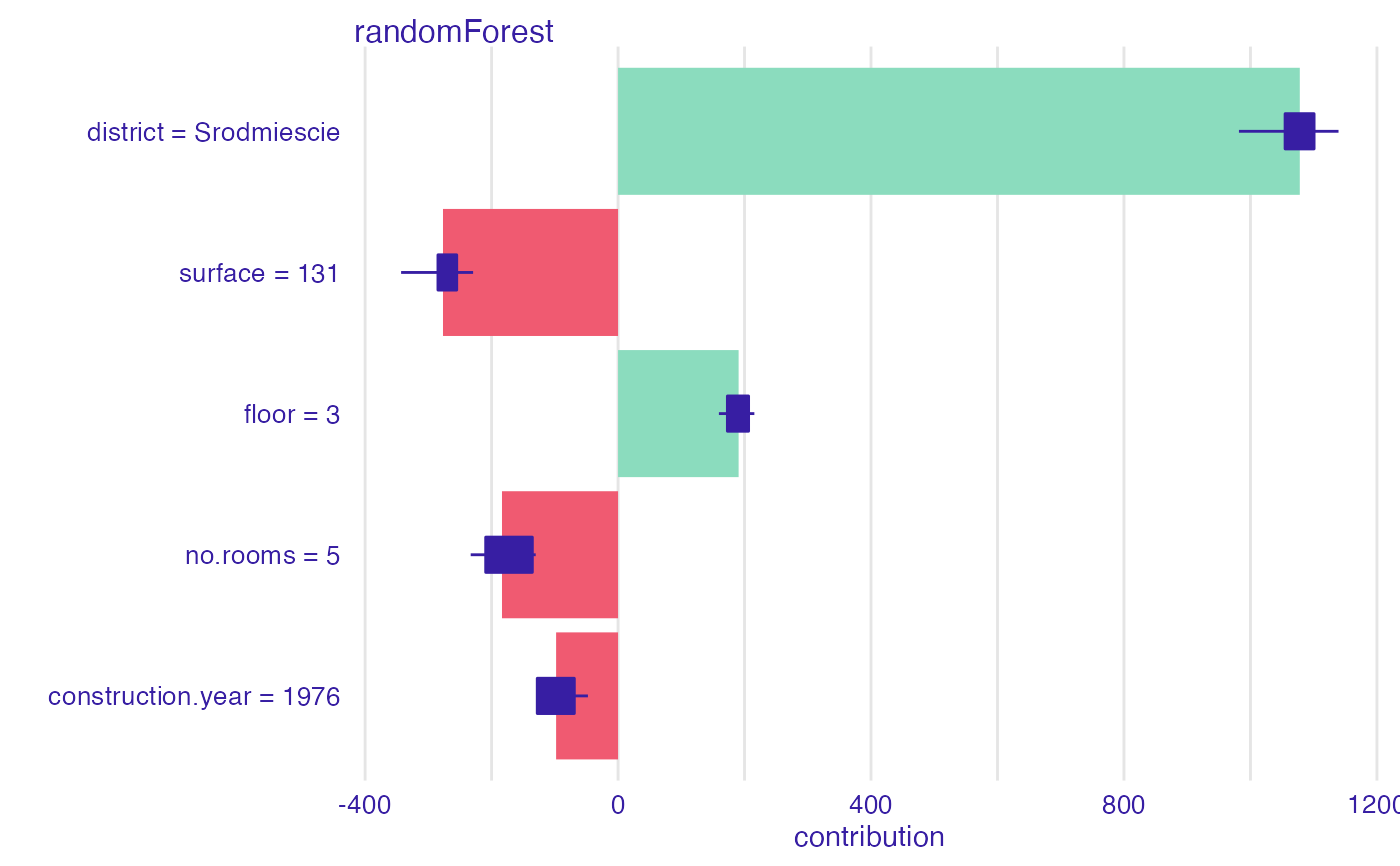# }